# Unsteady Heat and Mass Transfer Effects on an Impulsively Started Infinite Vertical Plate in the Presence of Porous Medium

Unsteady Heat and Mass Transfer Effects on an Impulsively Started Infinite Vertical Plate in the Presence of Porous Medium

Loganathan. P* Sivapoornapriya. C

Department of Mathematics, Anna University, Chennai 600025, India.

Corresponding Author Email:
logu@annauniv.edu, math.priya@gmail.com
Page:
69-74
|
DOI:
https://doi.org/10.18280/ijht.330211
N/A
|
Accepted:
N/A
|
Published:
30 June 2015
| Citation

OPEN ACCESS

Abstract:

The Heat and Mass transfer effects on an unsteady natural convective flow over an impulsively started vertical plate in the presence of porous medium is investigated. The dimensionless governing partial differential equations are solved by Laplace transform method. Exact analytical solutions satisfying the governing coupled partial differential equations and boundary conditions are obtained. The velocity, temperature and concentration, skin friction coefficient, Nusselt number and Sherwood number are studied for various physical parameters. It is observed that the velocity boundary layer increases with increasing values of permeability, thermal Grashof numer band mass Grashof number. Thermal boundary layer decreases with increasing values of Prandtl number. The concentration boundary layer decreases with increasing values of Schmidt number.

Keywords:

Unsteady, infinite, Vertical plate, Porous medium.

1. Introduction

The phenomenon of natural convective flow involving heat and mass transfer arises frequently in many engineering problems. In many industries, the simultaneous effects of heat and mass transfer are involved with transport phenomena. Flow through porous medium has numerous applications in all the fields. In recent decades, the flow caused by the two buoyancy effects over a vertical plate in the presence of porous medium is of great interest. Areas of applications includes purification of crude oil, the flow of ground water around hot intrusions, energy storage and recovery, chemical reactor engineering, cooling of nuclear reactors, space craft design, liquid metals fluid and power generation system.

Soundalgekar  presented an exact solution for the flow past an infinite vertical isothermal plate impulsively started in a viscous incompressible fluid. The effect of mass transfer was studied by Soundalgekar . Exact analytic solutions for free convection boundary layers on a heated vertical plate with lateral mass flux embedded in a saturated porous medium is presented by Magyari and Keller . Muthucumaraswamy et al.  studied the exact solution to the problem of flow past an impulsively started infinite vertical plate in the presence of uniform heat and mass flux with the help of Laplace transform technique.  Loganathan  analyzed the radiation effects on the flow past an impulsively started vertical plate with mass transfer by Laplace transform technique. An exact solution for mass transfer effects on flow past an impulsively started infinite vertical plate with constant mass flux was investigated by Das et al. .

Natural convection heat and mass transfer in a porous medium was discussed by Bejan and Khair . Raptis  applied the explicit finite difference scheme to study the unsteady free convective flow through a porous medium bounded by a semi-infinite vertical plate. Soundalgekar  analyzed viscous dissipative heat on the two dimensional unsteady free convective flow past an infinite vertical porous plate when the temperature oscillates in time and there is a constant suction at the plate. The vertical free convection boundary layer flow in porous media owing to combined Heat and mass transfer has been discussed by Bejan and Neild .

Kim  investigated the unsteady MHD convective flow of polar fluids past a vertical moving porous plate in a porous medium. Ganeswara Reddy and Bhaskara Reddy  studied the effects of variable viscosity and thermal conductivity on an unsteady MHD convective heat and mass transfer past a semi-infinite vertical porous plate and they applied perturbation technique and shooting method to get the solution.

Rama Chandra Prasad et al.  investigated the radiation and mass transfer effects on an unsteady MHD convection flow past a semi-infinite vertical permeable moving plate embedded in a porous medium with viscous dissipation. Loganathan and Golden Stepha  analysed the chemical reaction and radiation effects on a micropolar fluid past a continuously moving porous plate in steady state.

The present study focuses on heat and mass transfer effects on unsteady natural convective flow over an impulsively started vertical plate in the presence of porous medium. Laplace transform technique is used to solve the governing partial differential equations. The numerical results for velocity, temperature, concentration, skin friction, Nusselt number, Sherwood number are presented graphically. The results are presented graphically for various parameters such as Prandtl number, Schmidt number, thermal Grashof number, mass Grashof number and permeability parameter.

2. Mathematical Analysis

An unsteady, laminar, incompressible, natural convective flow over an impulsively started vertical plate in the presence of porous medium is considered. Let the x-axis be taken along the direction of the vertical plate and the y-axis is taken normal to the plate. Initially, it is assumed that the fluid and the plate are of the same temperature and concentration.  As time increases, the plate is given an impulsive motion in the vertical upward direction with a constant velocity $u_{0}$. Hence the temperature of the plate and concentration level near the plate are raised to $T_{w}^{\prime}$ and $c_{w}^{\prime}$ respectively. Under these assumptions the boundary layer equations are given by

$\frac{\partial u}{\partial t^{\prime}}=g \beta\left(T^{\prime}-T_{\infty}^{\prime}\right)+g \beta^{*}\left(c^{\prime}-c_{\infty}^{\prime}\right)+v \frac{\partial^{2} u}{\partial y^{2}}-\frac{v}{K^{\prime}} u$   (1)

$\frac{\partial T^{\prime}}{\partial t^{\prime}}=\alpha \frac{\partial^{2} T^{\prime}}{\partial y^{2}}$      (2)

$\frac{\partial c^{\prime}}{\partial t^{\prime}}=D \frac{\partial^{2} c^{\prime}}{\partial y^{2}}$     (3)

Initial and boundary conditions are given by

$t^{\prime} \leq 0, u=0, T^{\prime}=T_{\infty}^{\prime}, c^{\prime}=c_{\infty}^{\prime}$ for all $y$

$t^{\prime}>0, u=u_{0}, T^{\prime}=T_{w}^{\prime}, c^{\prime}=c_{w}^{\prime}$ at $y=0$

$u \rightarrow 0, T^{\prime} \rightarrow T_{\infty}^{\prime}, c^{\prime} \rightarrow c_{\infty}^{\prime}$ as $y \rightarrow \infty$       (4)

Equations (1)-(4) are written in the non-dimensional form by using the following quantities

$U=\frac{u}{u_{0}}, Y=\frac{y u_{0}}{v}, t=\frac{t^{\prime} u_{0}^{2}}{v}, \theta=\frac{T^{\prime}-T_{\infty}^{\prime}}{T_{w}^{\prime}-T_{\infty}^{\prime}}$

$C=\frac{c^{\prime}-c_{\infty}^{\prime}}{c_{w}^{\prime}-c_{\infty}^{\prime}}, \quad \operatorname{Pr}=\frac{v}{\alpha}, \quad S c=\frac{v}{D}, \quad \tau=\frac{v \tau^{\prime}}{\mu u_{0}^{2}}$

$K=\frac{K^{\prime} u_{0}^{2}}{v^{2}}, \quad G r=\frac{v g \beta\left(T_{w}^{\prime}-T_{\infty}^{\prime}\right)}{u_{0}^{3}}, G c=\frac{v g \beta^{*}\left(c_{w}^{\prime}-c_{\infty}^{\prime}\right)}{u_{0}^{3}}$     (5)

where u is  the velocity components in x direction, t is the time, g is the acceleration due to gravity, $u_{0}$ is the uniform velocity of the plate, $\theta$ is the temperature, $T_{w}^{\prime}$ and $T_{\infty}^{\prime}$ are the wall temperature and ambient temperature, $c^{\prime}$ is the concentration, $c_{w}^{\prime}$ is the concentration near the wall, $c_{\infty}^{\prime}$ is the species concentration in the fluid far away from the plate, $\alpha$ is the thermal diffusivity, D is the mass diffusivity , $K^{\prime}$ is the permeability of the porous medium, $\beta$ and $\beta^{*}$ are the thermal and concentration expansion coefficient, $v$ is the kinematic viscosity.

The governing equations and boundary conditions in dimensionless form is given by

$\frac{\partial U}{\partial t}=G r \theta \theta+G c \mathrm{C}+\frac{\partial^{2} U}{\partial Y^{2}}-\frac{U}{K}$      (6)

$\frac{\partial \theta}{\partial t}=\frac{1}{\operatorname{Pr}} \frac{\partial^{2} \theta}{\partial Y^{2}}$    (7)

$\frac{\partial C}{\partial t}=\frac{1}{S c} \frac{\partial^{2} C}{\partial Y^{2}}$     (8)

Initial and boundary condition in dimensionless forms are

$t \leq 0, U=0, \theta=0, C=0$ for all $Y$

$t>0, U=1, \theta=1, C=1$ at $Y=0$

$U \rightarrow 0, \theta \rightarrow 0, C \rightarrow 0$ as $Y \rightarrow \infty$     (9)

where U is the non-dimensional velocity, T is the non-dimensional temperature, C is the non-dimensional concentration, Pr is the Prandtl number, Sc is the Schmidt number, K is the non-dimensional permeability parameter, Gr is the thermal Grashof number and Gc is the mass Grashof number.

3. Analytical Solution

The Laplace transform technique is used to get the analytical solution for the problem. Thus the governing equations in (6) - (8) reduces to the ordinary differential equations as follows

$\frac{d^{2} \bar{U}}{d Y^{2}}-\left(s+\frac{1}{K}\right) \bar{U}=-G r \bar{\theta}-G c \bar{C}$   (10)

$\frac{d^{2} \bar{\theta}}{d Y^{2}}-s \operatorname{Pr} \bar{\theta}=0$    (11)

$\frac{d^{2} \bar{C}}{d Y^{2}}-s S c \bar{C}=0$    (12)

Subject to the conditions

$t>0, \bar{U}=\frac{1}{s}, \bar{\theta}=\frac{1}{s}, \bar{C}=\frac{1}{s}$ at $\mathrm{Y}=0$

$\bar{U} \rightarrow 0, \bar{\theta} \rightarrow 0, \bar{C} \rightarrow 0$ as $Y \rightarrow \infty$    (13)

Solving these equations with respect to the conditions in eqn. (13), we get

$\bar{C}=\frac{1}{s} e^{-\sqrt{s c s}} Y$   (14)

$\bar{\theta}=\frac{1}{s} e^{-\sqrt{\operatorname{Pr} s} Y}$     (15)

\begin{aligned} \bar{U}=(&\left.\frac{1}{s}-\frac{a_{1}}{s\left(s-b_{1}\right)}-\frac{a_{2}}{s\left(s-b_{2}\right)}\right) e^{-\sqrt{s+\frac{1}{k}} Y} +\frac{a_{1}}{s\left(s-b_{1}\right)} e^{-\sqrt{s \operatorname{Pr}} Y}+\frac{a_{2}}{s\left(s-b_{2}\right)} e^{-\sqrt{s S c}Y} \end{aligned}   (16)

where

$a_{1}=\frac{-G r}{\operatorname{Pr}-1}, a_{2}=\frac{-G c}{S c-1}$

$b_{1}=\frac{1}{K(\mathrm{Pr}-1)}, b_{2}=\frac{1}{K(S c-1)}$

Then taking the inverse Laplace transform for the equations (14) – (16), we get the concentration, temperature and velocity as follows

$C=\operatorname{erfc}(\eta \sqrt{S c})$     (17)

$\theta=\operatorname{erfc}(\eta \sqrt{\operatorname{Pr}})$      (18)

$U=\left(\frac{1}{2}+\frac{a_{1}}{2 b_{1}}+\frac{a_{2}}{2 b_{2}}\right)\left[\begin{array}{c}e^{-2 \eta \sqrt{L_{1}}} \operatorname{erfc}(\eta-\sqrt{L_{1}}) \\ +e^{2 \eta \sqrt{L_{1}}} \operatorname{erfc}(\eta+\sqrt{L_{1}})\end{array}\right]$

$-\frac{a_{1} e^{b_{1} t}}{2 b_{1}}\left[e^{-2 \eta \sqrt{L_{2}}} \operatorname{erfc}(\eta-\sqrt{L_{2}})+e^{2 \eta \sqrt{L_{2}}} \operatorname{erfc}(\eta+\sqrt{L_{2}})\right]$

$-\frac{a_{1}}{b_{1}} \operatorname{erfc}(\eta \sqrt{\operatorname{Pr}})-\frac{a_{2}}{b_{2}} \operatorname{erfc}(\eta \sqrt{S c})$

$-\frac{a_{2} e^{b_{2} t}}{2 b_{2}}\left[e^{-2 \eta \sqrt{L_{3}}} \operatorname{erfc}(\eta-\sqrt{L_{3}})+e^{2 \eta \sqrt{L_{3}}} \operatorname{erfc}(\eta+\sqrt{L_{3}})\right.$

$+\frac{a_{1} e^{b_{1} t}}{2 b_{1}}\left[\begin{array}{c}e^{2 \eta \sqrt{\mathrm{Pr} b_{1} t}} \operatorname{erfc}(\eta \sqrt{\mathrm{Pr}}+\sqrt{b_{1} t}) \\ +e^{-2 \eta \sqrt{\operatorname{Pr} b_{1} t}} \operatorname{erfc}(\eta \sqrt{\operatorname{Pr}}-\sqrt{b_{1} t})\end{array}\right]$

$+\frac{a_{2} e^{b_{2} t}}{2 b_{2}}\left[\begin{array}{c}e^{2 \eta \sqrt{S c b_{2} t}} \operatorname{erfc}(\eta \sqrt{S c}+\sqrt{b_{2} t}) \\ +e^{-2 \eta \sqrt{S c b_{2} t}} \operatorname{erfc}(\eta \sqrt{S c}-\sqrt{b_{2} t})\end{array}\right]$  (19)

where

$\eta=\frac{Y}{2 \sqrt{t}}, L_{1}=\frac{t}{K}$

$L_{2}=\left(b_{1}+\frac{1}{K}\right) t, \quad L_{3}=\left(b_{2}+\frac{1}{K}\right) t$

The expressions in equations (17) – (19) are the general solution for the problem. Knowing the velocity field, it is interesting to study the effects of permeability of the porous medium, thermal Grashof number, mass Grashof number, Prandtl number and Schmidt number on skin-frition. The skin-friction is given by

$\tau^{\prime}=-\mu\left(\frac{\partial u}{\partial y}\right)_{y=0}$  (20)

By virtue of equation (5), equation (20) reduces to

$\tau=-\left(\frac{\partial U}{\partial Y}\right)_{Y=0}$     (21)

$\tau=-\left(\begin{array}{c}-\left(1+\frac{a_{1}}{b_{1}}+\frac{a_{2}}{b_{2}}\right) \frac{1}{\sqrt{t \pi}}\left[e^{-L_{1}}+\sqrt{L_{1} \pi} \operatorname{erf}(\sqrt{L_{1}})\right] \\ +\frac{a_{1} e^{b_{1} t}}{b_{1} \sqrt{t \pi}}\left[e^{-L_{2}}+\sqrt{L_{2} \pi} \operatorname{erf}(\sqrt{L_{2}})\right] \\ +\frac{a_{2} e^{b_{2} t}}{b_{2} \sqrt{t \pi}}\left[e^{-L_{3}}+\sqrt{L_{3} \pi} \operatorname{erf}(\sqrt{L_{3}})\right] \\ -\frac{a_{1} \sqrt{\operatorname{Pr}} e^{b_{1} t}}{b_{1} \sqrt{t \pi}}\left[e^{-b_{1} t}+\sqrt{b_{1}} \operatorname{erf}(\sqrt{b_{1} t})\right] \\ -\frac{a_{2} \sqrt{\operatorname{Sc}} e^{b_{2} t}}{b_{2} \sqrt{t \pi}}\left[e^{-b_{2} t}+\sqrt{b_{2}} \operatorname{erf}(\sqrt{b_{2} t})\right]\end{array}\right)$     (22)

By knowing the temperature distribution, it is interesting to learn about the rate of heat transfer, which depends on time and the Prandtl number. The Nusselt number is given by

$N u=-\left(\frac{\partial \theta}{\partial Y}\right)_{Y=0}$    (23)

$N u=\frac{\sqrt{\operatorname{Pr}}}{\sqrt{\pi t}}$     (24)

By knowing the concentration distribution it is customary to study the rate of mass transfer. The rate of mass transfer which depends on time and Schmidt number is given by

$S h=-\left(\frac{\partial C}{\partial Y}\right)_{Y=0}$     (25)

$S h=\frac{\sqrt{S c}}{\sqrt{\pi t}}$     (26)

4. Results and Discussion

In order to get the physical insight of the problem, the velocity, temperature, concentration, skin-friction, Nusselt number and Sherwood number are computed for various values of Prandtl number, Schmidt number, permeability, thermal Grashof number and mass Grashof number. The values of Prandtl number is chosen in such a way that, it represents air (Pr = 0.73), water (Pr = 7) and methanol (Pr = 11.62). For species concentration, real gases are considered. For this purpose the values of Schmidt number Sc are chosen to represent various species at low concentration in air. Schmidt numbers under consideration are Hydrogen (Sc = 0.16), water vapor (Sc = 0.60), Ammonia (Sc=0.78), Carbondioxide (Sc=0.94) and Ethyl benzene (2). .

The numerical results are shown graphically. In Figures (1) – (4), the velocity profile for various values of permeability of the porous medium (K), Prandtl numbers (Pr), Schmidt number (Sc), time (t) are presented. It is observed that the velocity increases with increasing values of K, and t. Because of the Darcy’s law which relates the flow rate and fluid properties to the pressure gradient applied to the porous medium there is an increase in the velocity boundary layer for increasing values of Permeability. But a reverse process is seen for Pr and Sc. The velocity boundary layer decreases with increasing values of Sc and Pr. Since the velocity is coupled with temperature and concentration, the effects of Prandtl number and Schmidt number are easily seen in the velocity profile. In physical aspect, the velocity profile for air (Pr = 0.73) is heavier than the water (Pr = 7). It is also seen that an increase in the Schmidt number leads to a fall in the velocity of air.

In Figure (5), the velocity profile for various values of thermal Grashof number (Gr) and mass Grashof number (Gc) are plotted for the cases heating (Gr<0, Gc<0) and cooling (Gr>0, Gc>0)of the plate. The heating and cooling takes place by setting up natural current due to temperature and concentration gradients. It is concluded from the figure that the velocity boundary layer increases with increasing values of thermal Grashof number and mass Grashof number for cooling of the plate and a reverse phenomenon is observed in the heating of the plate.

## 1.jpg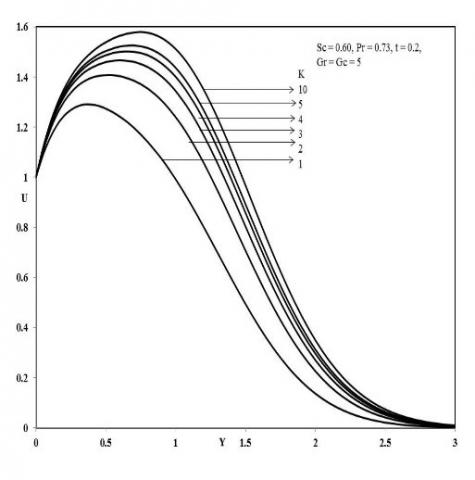Figure 1. Velocity profile for different values of K

## 2.jpg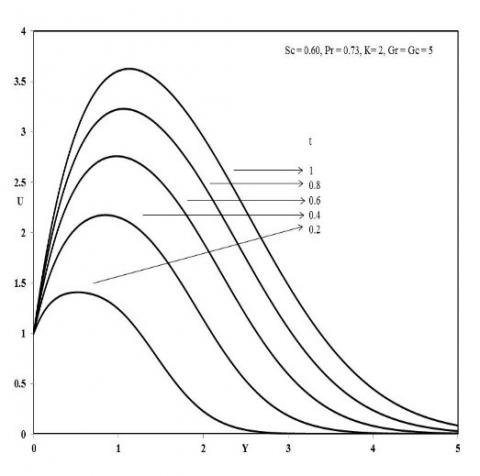Figure 2. Velocity profile for different values of t

## 3.jpg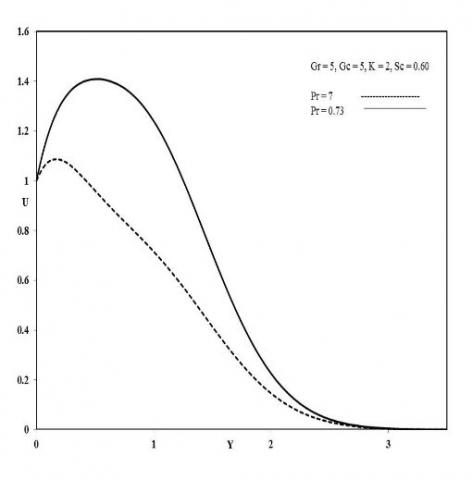Figure 3. Velocity profile for different values of Pr

Figure (6) and (7), depicts the temperature profile and concentration profile against various values of time. It is clearly seen that the thermal boundary layer gradually increases with gradual increase of time t. The same type of behavior is seen for concentration profile.The concentration profile increases with increasing time.

## 4.jpg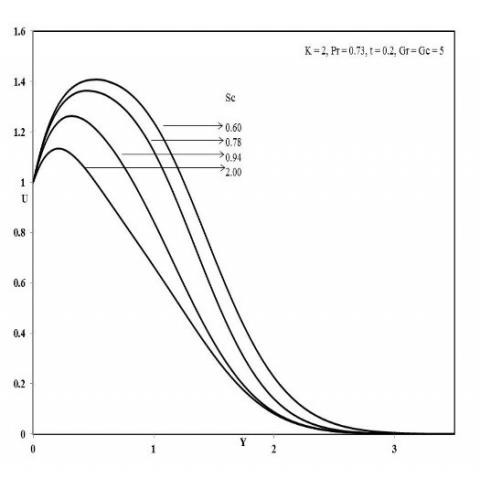Figure 4. Velocity profile for different values of Sc

## 5.jpg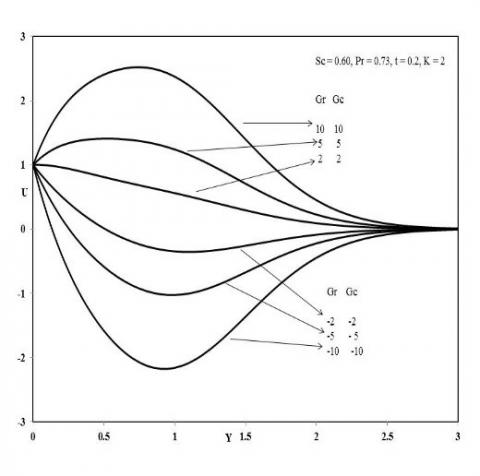Figure 5. Velocity profile for different values of Gr and Gc

## 6.jpgFigure 6. Temperature profile for different values of t

Figure (8), depicts the temperature profile for various values of Prandtl number.For increasing values of Prandtl number the thermal boundary layer decreases. As the Prandtl number is the characteristics of the relationship between the momentum boundary layer thickness to thermal boundary layer thickness, the thermal boundary layer becomes thin by increasing the Prandtl number. Hence there is a decrease in the thermal boundary layer thickness.

Figure (9), depicts the concentration boundary layer profile for various values of Sc. Schmidt number being the ratio between the momentum diffusivity to species diffusivity. It physically relates the thickness of the viscous boundary layer and concentration boundary layer. Thus the concentration boundary layer decreases with increasing values of Sc. This is due to the fact that a large Sc corresponds to a concentration boundary layer relative to the momentum boundary layer.

In Figure (10), Skin-friction for various values of Sc is presented. Skin-friction increases with decreasing values of Sc in the presence of air (Pr = 0.73). An increase in Sc decelerates the velocity of the flow. This deceleration in the velocity of the flow reduces the shear stress along the wall which leads to a decrease in the values of the skin-friction.

## 7.jpgFigure 7. Concentration profile for different values of t

## 8.jpg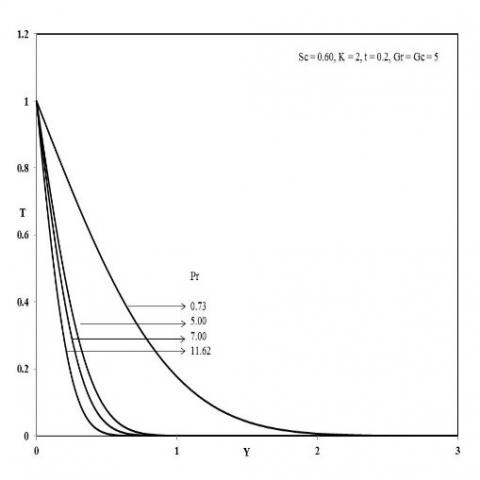Figure 8. Temperature profile for different values of Pr

## 9.jpg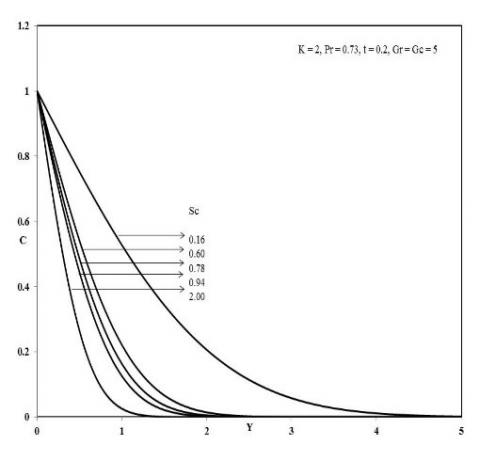Figure 9. Concentration profile for different values of Sc

In Figure (11), an increase in Prandtl number increases the Nusselt number. Due to pure conduction process the rate of heat transfer is not seen initially. Later the heat transfer rate increase with increasing Prandtl number. In physical aspect the rate of heat transfer for air (Pr = 0.73) is lesser when compared to water (Pr = 7).

Figure (12), depicts that for increasing values of Schmidt number, the values of Sherwood number increases and decreases with time for fixed Grashof number and Prandtl number. Physically saying the mass transfer rate is induced for increase in the species concentration.

## 10.jpg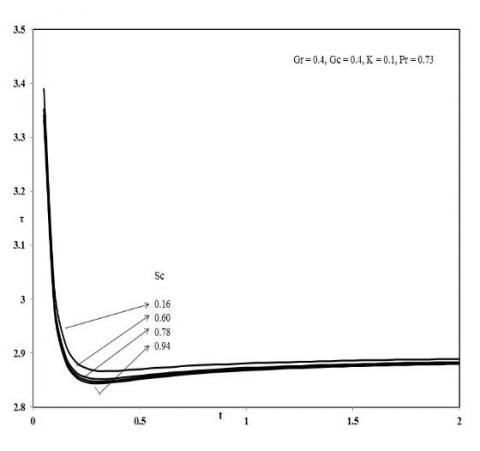Figure 10. Skin-friction for different values of Sc

## 11.jpgFigure 11. Nusselt number for different values of Pr

## 12.jpgFigure 12. Sherwood number for different values of Sc

5. Conclusion

The present study deals with an unsteady natural convective flow past an impulsively started vertical plate in the presence of porous medium. The analytical study focuses on the behavior of velocity, temperature, concentration with various parameters like Prandtl number, Schmidt number, thermal Grashof number, mass Grashof number and the permeability of the porous medium. Also the shear stress, Nusselt number and Sherwood number are discussed for various parameters. Hence it is concluded that

► The velocity profile increases with increasing values of K, Gr, Gc, t. But decreases for Pr and Sc.

► Thermal boundary layer increases for increasing values of t and decreases for increasing values of Pr.

► Concentration boundary layer increases for increasing values of t and decreases for increasing values of Sc.

► Skin-friction decreases and Sherwood number increases for increasing values of Schmidt number.

► Nusselt number increases with increasing values of Prandtl number.

Nomenclature
 $u$ velocity component in x – direction[Lt-1] $t$ time [t] $g$ acceleration due to gravity[Lt-2] $\mathrm{u}_{0}$ uniform velocity of the plate[Lt-1] $\theta$ temperature[t] $T_{w}^{\prime}$ wall temperature[T] $T_{\infty}$ ambient temperature[T] $c^{\prime}$ concentration[ML-3] $c_{w}^{\prime}$ wall concentration[ML-3] $c_{\infty}^{\prime}$ ambient concentration[ML-3] $D$ mass diffusivity[L2t-1] $K^{\prime}$ Permeability[L2] Greek Symbols α thermal diffusivity[L2t-1] $\beta$ thermal expansion coefficient[T-1] $\beta^{*}$ concentration expansion coefficient[M-1L3] Sub Scripts w plate wall $\infty$ ambient
References

1.  V.  M.  Soundalgekar,  Free  convection  effects  on  the Stokes problem for a vertical plate, ASME J. Heat Transfer, vol. 99, pp. 499–501, 1977.

2.  V. M. Soundalgekar, Effects of mass transfer and free convection currents on the flow past an impulsively started vertical plate, ASME J. Appl. Mech., vol. 46, pp. 757–760, 1979.

3.  E. Magyari and B. Keller, Exact analytic solutions for free convection  boundary layers on  a heated  vertical plate with lateral mass flux embedded in a saturated porous medium. Heat and Mass transfer. vol. 36, pp.109-116, 2000.

4. R.   Muthukumaraswamy,   P.   Ganesan   and   V.   M. Soundalgekar, Heat and mass transfer effects on flow past an impulsively started vertical plate. Acta Mechanica. vol. 146, pp. 1-8, 2001.

5. P. Loganatha and P. Ganesan, Effects of radiation on flow past an impulsively started vertical plate with mass transfer. Journal of Engineering Physics and Thermophysics, vol. 79(1), pp. 65-72, 2006.

6. U. N. Das, S. N. Ray and V. M. Soundalgekar, Mass transfer  effects  on  flow  past  an  impulsively  started infinite vertical plate with constant mass flux – an exact solution. Heat and Mass Transfer. vol. 31, pp. 163-167, 1996.

7.  A.  Bejan,  and  R.  Khair,  Heat  and  mass  transfer  by natural  convection  in  a  porous medium.  Int. J.  Heat Mass Transfer. vol. 28, pp. 909-918, 1985.

8. A.  Raptis,  A.  K.  Singh  K.  D.  Rai,  Finite difference analysis of unsteady free convective flow through a porous medium adjacent to semi-infinite vertical plate. Mechanics Research Communications, vol.14, pp. 9-16, 1987.

9.  V.  M.  Soundalgekar,  Free  convection  effects  on  the oscillatory flow past an infinite vertical, porous plate with constant suction. Proc. R. Soc. Lond. A. vol. 333, pp. 25-36, 1973.

10. D. A. Neild, and A.  Bejan, Convection in porous media, Springer Verlag, 1992.

11. Y. J. Kim, Unsteady MHD convection  flow of polar fluids past a vertical moving porous plate in a porous medium. Int.  J. of Heat and Mass Transfer. vol. 44, pp. 2791-2799, 2001.

12. M.  Ganaeswara  Reddy and  N.  Bhaskar  Reddy, Unsteady MHD convective heat and mass transfer past a semi-infinite vertical porous plate with variable viscosity and thermal conductivity. Int. J. of App. Math. and Comp. vol. 1, pp. 104-117, 2009.

13.  V.   Ramachandra   Prasad   and   N.   Bhaskar   Reddy, Radiation  and  mass  transfer  effects  on  an  unsteady MHD convection flow past a semi-infinite vertical permeable moving plate embedded in a porous medium with viscous dissipation. Indian Journal of Pure & Applied Physics. vol. 46, pp. 81-92, 2008.

14. P.   Loganathan   and   N.   Golden   Stepha,   Chemical reaction effects on flow of micropolar fluid past a continuously moving porous plate in the presence of radiation, Int. J. of Heat & Tech., vol. 30, 2, pp. 107-113, 2012.